# Revision Notes For CBSE Class 10 Maths Chapter 3 Pair of Linear Equations in Two Variables

In chapter 3 of class 10 maths, students are introduced to several methods of solving linear equation algebraically as well as graphically. They will also learn about other methods like substitution method, elimination method and cross multiplication methods. While there are many topics to cover and understand, free CBSE revision notes for class 10 maths chapter 3 – pair of linear equations in two variables is available here with detailed explanations to further make things easy for students. These notes offer the necessary insight into how the math problems should be solved. Students can study smartly with these notes and at the same time be well prepared to answer any type of question that may be asked in the examinations.

Take a glimpse of class 10 maths chapter 3 CBSE revision notes PDF below.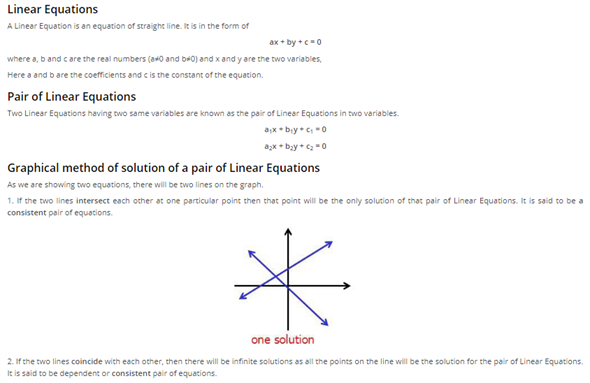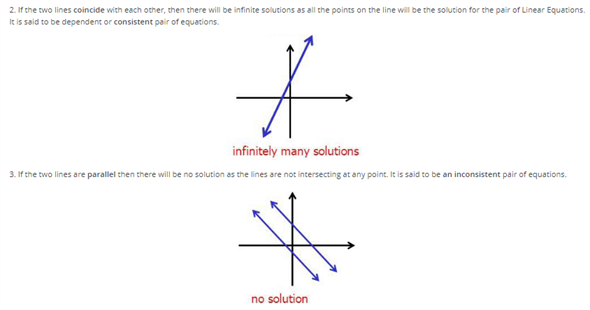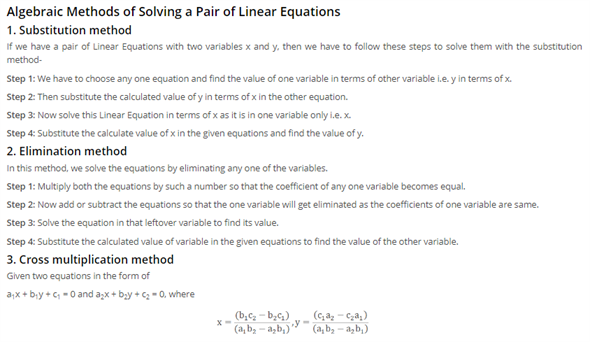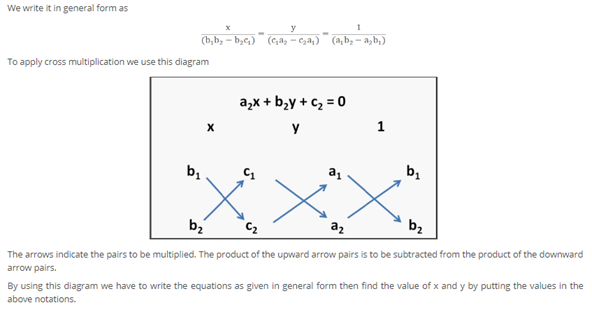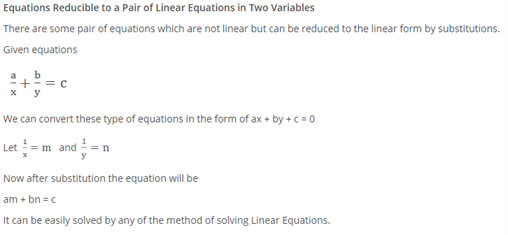Access CBSE Class 10 Maths Sample Papers Here.

Access NCERT Class 10 Maths Book Here.